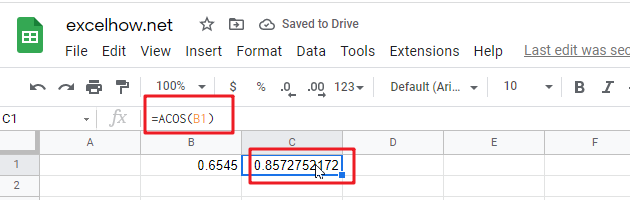# ExcelHow

This post will guide you how to use Google Sheets ACOS function with syntax and examples.

## Description

The Google Sheets ACOS function returns the arccosine value of a number.

The ACOS function can be used to get the inverse cosine of a number in google sheets. The purpose of this function is to get the inverse cosine of a given value and its returned value is angle in radians.

The ACOS function is a build-in function in Google Sheets and it is categorized as a MATH function.

## Syntax

The syntax of the ACOS function is as below:

= ACOS(number)

Where the ACOS function argument is:

• Number – This is a required argument.  A number between -1 and 1 that you want the arccosine value.

## Google Sheets ACOS Function Examples

The below examples will show you how to use google sheets ACOS function to get the inverse cosine of a value.

#1 =ACOS(B1)Note: the above formula will return the arccosine value of B1 cell.